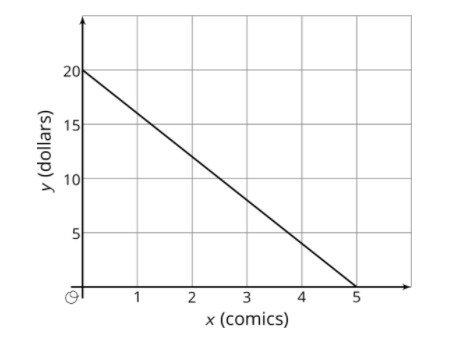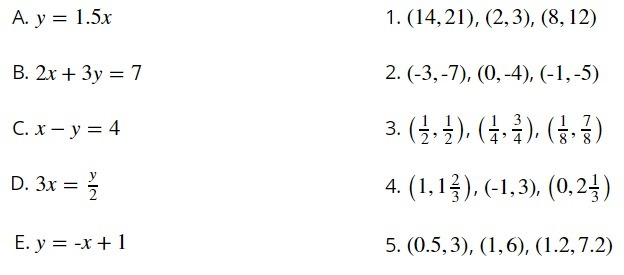PSABM5WP - Practice---Acc7.5 Lesson 10 Solutions to Linear Equations (8.EE.B, 8.EE.C)

Select all of the ordered pairs (x,y) that are solutions to the linear equation 236.

Select all that apply:
Part A)

The graph shows a linear relationship between x and y.x represents the number of comic books Priya buys at the store, all at the same price, and represents the amount of money (in dollars) Priya has after buying the comic books.

What is the x-intercept of this line?

Type your answer below as a number (example: 5, 3.1, 4 1/2, or 3/2):
Part B)

What is the y-intercept of this line?

Type your answer below as a number (example: 5, 3.1, 4 1/2, or 3/2):
Part C)

Interpret the x- and y-intercepts of this line.

Part D)

Find the slope of this line.

Type your answer below as a number (example: 5, 3.1, 4 1/2, or 3/2):
Part E)

Interpret the slope of this line.

Part F)

Find the equation for this line.

Complete the equation below:

y = _______________

Part G)

If Priya buys 3 comics, how much money will she have remaining?

Do not include units ($) in your answer. Type your answer below as a number (example: 5, 3.1, 4 1/2, or 3/2): Part A) Match each equation on the left with its three solutions on the right.For each letter, your answer should be the just the number of the corresponding solutions on the right. For example: If equation A matches with the three solutions of option number 3 on the right, your answer would be 3. A: copied for free from openupresources.org Select one: Part B) B: Select one: Part C) C: Select one: Part D) D: Select one: Part E) E: Select one: Part A) A container of fuel dispenses fuel at the rate of 5 gallons per second. If represents the amount of fuel remaining in the container, and x represents the number of seconds that have passed since the fuel started dispensing, then x and y satisfy a linear relationship. In the coordinate plane, will the slope of the line representing that relationship have a positive, negative, or zero slope? copied for free from openupresources.org Select one: Part B) Explain how you know. Type your answer below: Part A) A sandwich store charges a delivery fee to bring lunch to an office building. One office pays$33 for 4 turkey sandwiches. Another office pays $61 for 8 turkey sandwiches. How much does each turkey sandwich add to the cost of the delivery? Do not include units ($) in your answer.